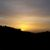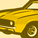QlikView App Dev

Discussion Board for collaboration related to QlikView App Development.

Announcements
QLIKWORLD LIVE! MAY 16 - 19TH, EARLY BIRD DISCOUNTS! REGISTER TODAY
cancel
Showing results for
Did you mean:Partner

Summing a value up to Selected Year

Hi,

I've created a Pivot Chart.  I need to sum all values in a field up to selected year for the selected dimension.

For example, if user selects year 2017, the values in field Inception should be summed up for years 2016 and 2017, assuming available data starts in year 2016.  If user selected year 2018, values in field Inception should be summed up starting in 2016, 2017 and 2018; etc.

Sample data in attached file.

1 Solution

Accepted SolutionsMVP

Assuming you have Year field available.

Create variable vSelectedYear  and put below in value

=max(Year)

In Pie chart, put expression like below

= sum({<Year ={"<=\$(vSelectedYear)"}>}Sales)

5 RepliesMVP

Assuming you have Year field available.

Create variable vSelectedYear  and put below in value

=max(Year)

In Pie chart, put expression like below

= sum({<Year ={"<=\$(vSelectedYear)"}>}Sales)Partner
Author

Not working. 😞

I've tried all of these options, but none have worked.

=sum({<FiscalYear={"<=\$(vSelectedYear)"}>}Inception)
=sum({<FiscalYear={"<=\$(=max(FiscalYear))"}>}Inception)
=Sum({<FiscalYear={">=\$(=Min(FiscalYear))<=\$(=Max(FiscalYear)+1)"}>}Inception)Creator II

Hi,

Try the below expression.

=Sum({<FiscalYear={">=\$(=Min({1}FiscalYear))<=\$(=Max(FiscalYear))"}>}Inception)

Thanks,

JeshMVP

what is the format of FiscalYear? Make sure it is number format. Try to check max(FiscalYear) value in text object or KPI object(qlik sense)Partner
Author

Hi, I was using the year field in the calendar table for the Years List Box.  Now I'm using the Year field in the fact table, which works precisely.

Thanks!Community Browser